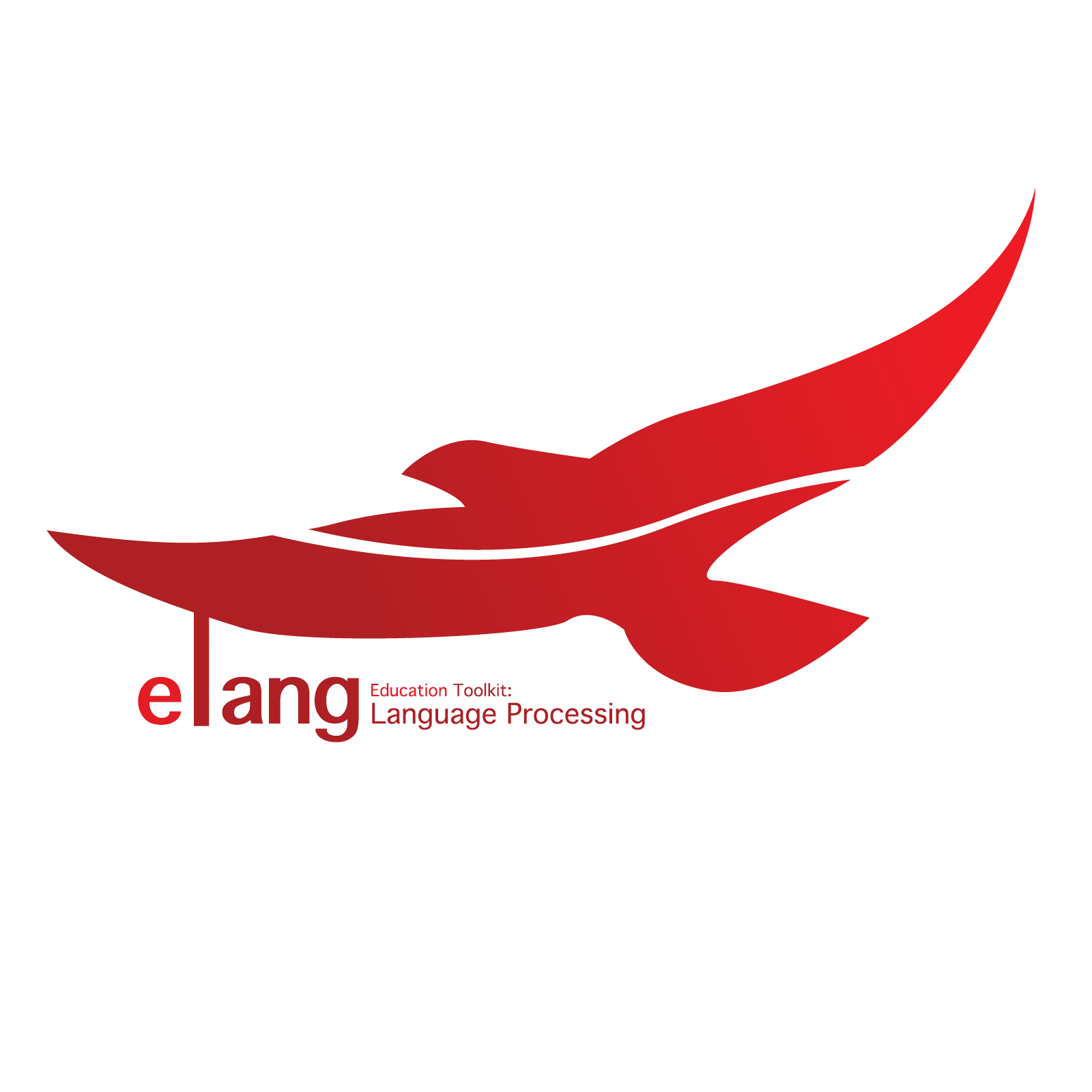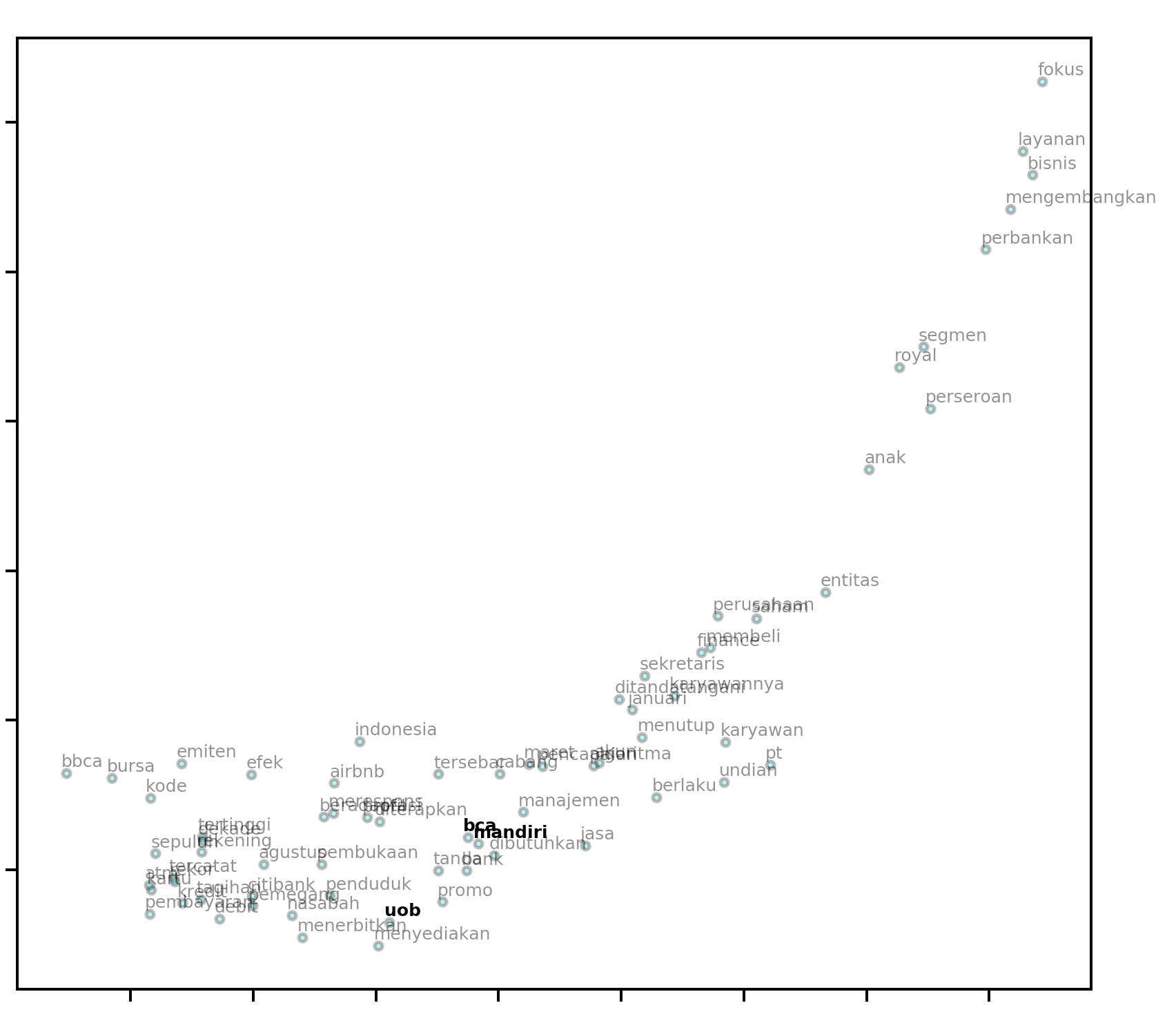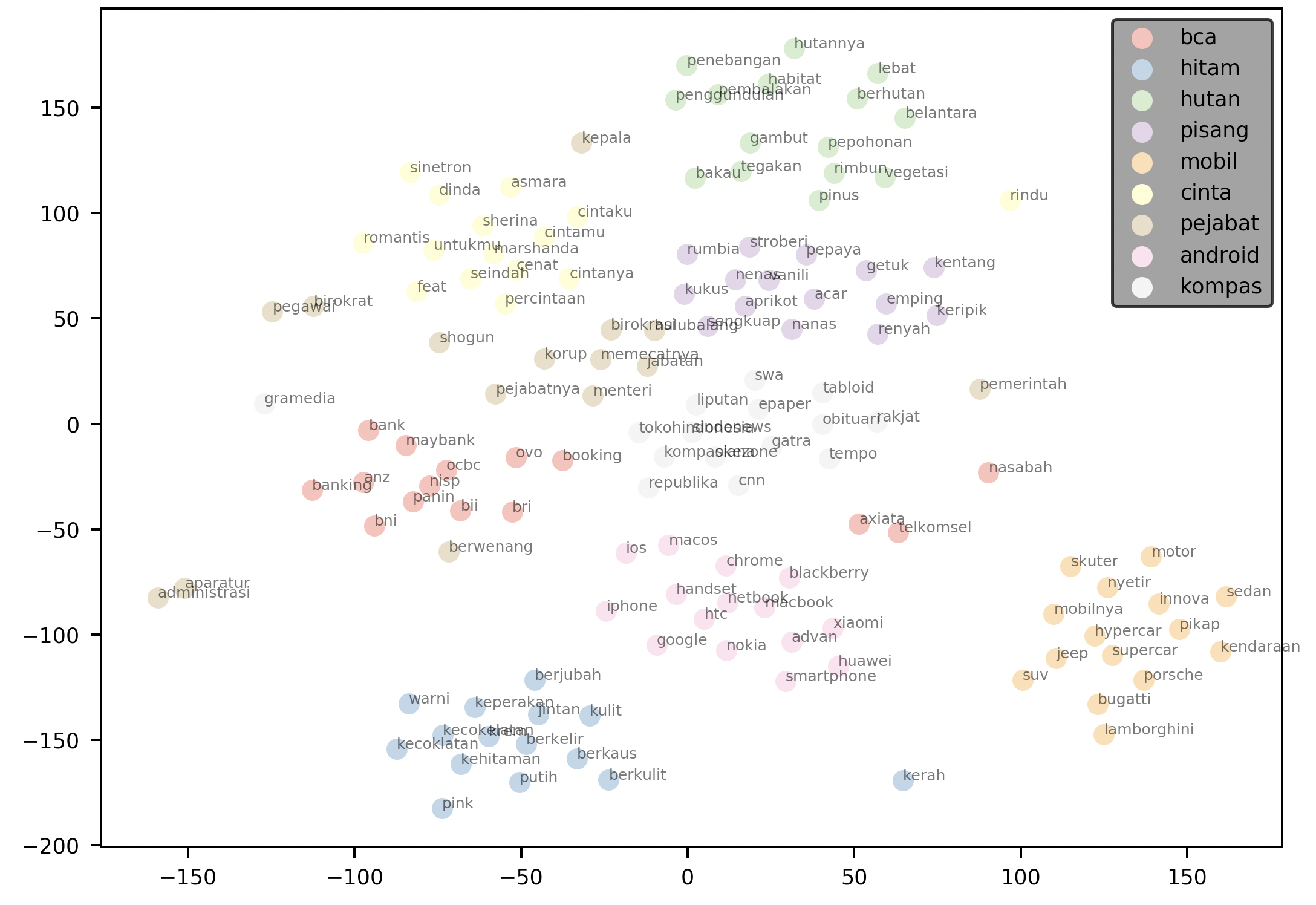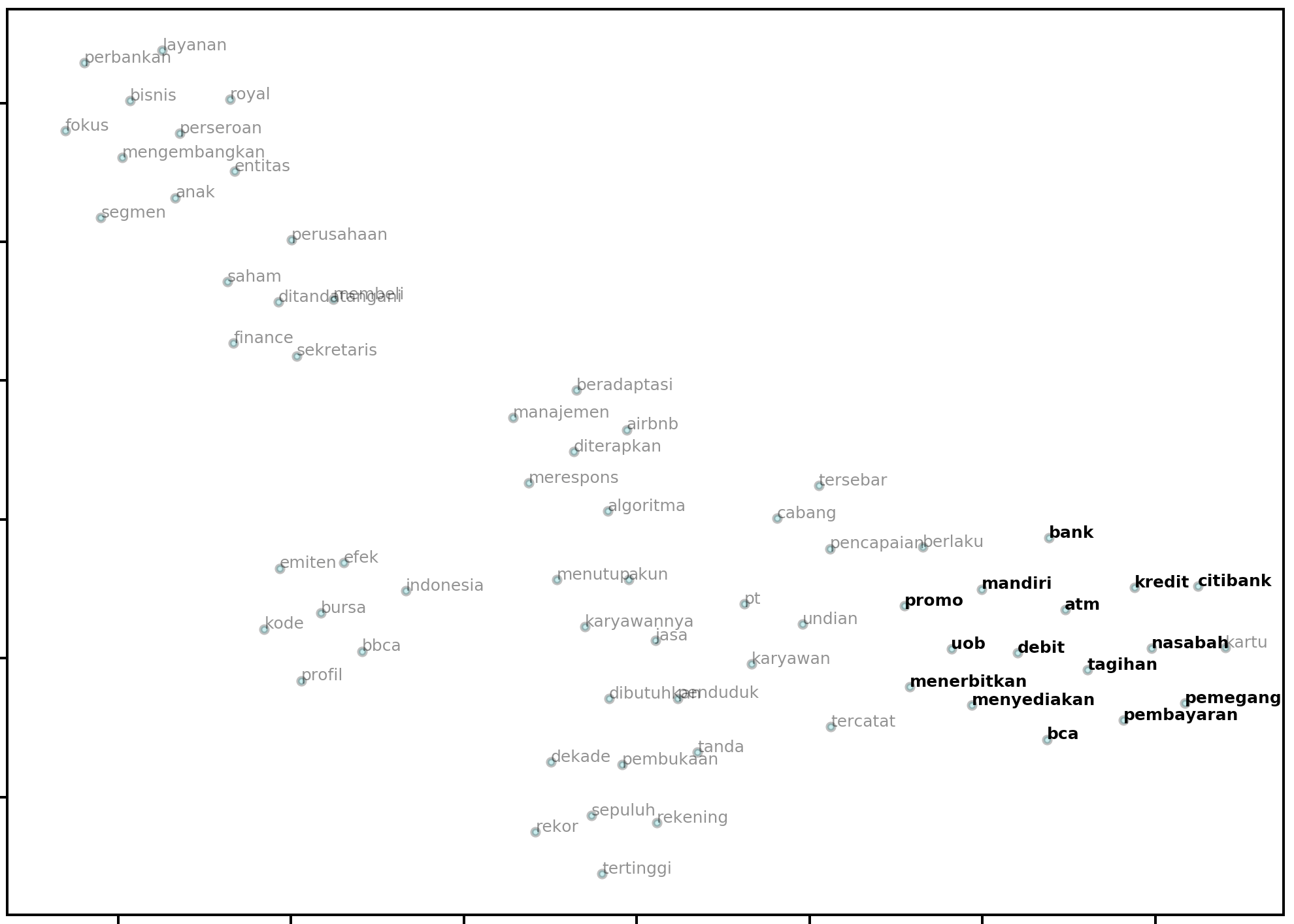Word Embedding(E) utilities for Language(Lang) Models

# Word Embedding utilities for Language Models

Elang is an acronym that combines the phrases Embedding (E) and Language (Lang) Models. Its goal is to help NLP (natural language processing) researchers, Word2Vec practitioners, educators and data scientists be more productive in training language models and explaining key concepts in word embeddings.

Key features as of the 0.1 release can be grouped as follow:

• Corpus-building utility

• build_from_wikipedia_random: Build English / Indonesian corpus using random articles from Wikipedia
• build_from_wikipedia_branch: Build English / Indonesian corpus by building a "topic branch" off Wikipedia
• Text processing utility

• remove_stopwords_id: Remove stopwords (Indonesian)
• remove_region_id: Remove region entity (Indonesian)
• remove_calendar_id: Remove calendar words (Indonesian)
• remove_vulgarity_id: Remove vulgarity (Indonesian)
• Embedding Visualization Utility (see illustration below)

• plot2d: 2D plot with emphasis on words of interest
• plotNeighbours: 2D plot with neighbors of words## Elang

Elang also means "eagle" in Bahasa Indonesia, and the elang Jawa (Javan hawk-eagle) is the national bird of Indonesia, more commonly referred to as Garuda.

The package provides a collection of utility functions and tools that interface with gensim, matplotlib and scikit-learn, as well as curated negative lists for Bahasa Indonesia (kata kasar / vulgar words, stopwords etc) and useful preprocesisng functions. It abstracts away the mundane task so you can train your Word2Vec model faster, and obtain visual feedback on your model more quickly.

# Quick Demo

### 2-d Word Embedding Visualization

Install the latest version of elang:

pip install --upgrade elang


Performing word embeddings in 2 lines of code gets you a visualization:

from elang.plot.utils import plot2d
from gensim.models import Word2Vec

plot2d(model)
# output:It even looks like a soaring eagle with its outstretched wings!

### Visualizing Neighbors in 2-dimensional space

elang also includes visualization methods to help you visualize a user-defined k number of neighbors to each words. When draggable is set to True, you will obtain a legend that you can move around in the resulting plot.

words = ['bca', 'hitam', 'hutan', 'pisang', 'mobil', "cinta", "pejabat", "android", "kompas"]

plotNeighbours(model,
words,
method="TSNE",
k=15,
draggable=True)The plot above plots the 15 nearest neighbors for each word in the supplied words argument. It then renders the plot with a draggable legend.

### Scikit-Learn Compatability

Because the dimensionality reduction procedure is handled by the underlying sklearn code, you can use any of the valid parameters in the function call to plot2d and plotNeighbours and they will be handed off to the underlying method. Common examples are the perplexity, n_iter and random_state parameters:

model = Word2Vec.load("path.to.model")
bca = model.wv.most_similar("bca", topn=14)
similar_bca = [w for w in bca]
plot2d(
model,
method="PCA",
targets=similar_bca,
perplexity=20,
early_exaggeration=50,
n_iter=2000,
random_state=0,
)


Output:### Building a Word2Vec model from Wikipedia

from elang.word2vec.builder import build_from_wikipedia
# a convenient wrapper to build_from_wikipedia_random or build_from_wikipedia_branch
model1 = build_from_wikipedia(n=3, lang="id")
model2 = build_from_wikipedia(slug="Koronavirus", lang="id", levels=2)
print(model1)
# returns: Word2Vec(vocab=190, size=100, alpha=0.025)


The code above constructs two Word2Vec models, model1 and model2. The function that constructs these models does so by building a corpus from 3 (n) random articles on id.wikipedia.org (id). The corpus can optionally be saved by passing the save=True argument to the function call.

In model2, the function starts off by looking at the article: https://id.wikipedia.org/wiki/Koronavirus (determined by id and slug), and then find all related articles (level 1), and subsequently all related articles to those related articles (level 2). A corpus is built using all articles it find along this search branch (levels).

#### Building a Corpus from Wikipedia (without Word2Vec model)

If you would like to build a corpus, but not have the function return a Word2Vec model, simply pass model=False and save=True. The save argument will create a /corpus directory and save the corpus in a .txt file.

build_from_wikipedia(n=10, lang="en", save=True)


The function call above will create a Corpus from the international (english) version of Wikipedia and save it to the following file in your working directory: corpus/wikipedia_random_10_en.txt

## Project details

This version0.1.10.1.00.0.110.0.100.0.90.0.9.dev1 pre-release0.0.80.0.60.0.50.0.40.0.3

Uploaded source
Uploaded py3